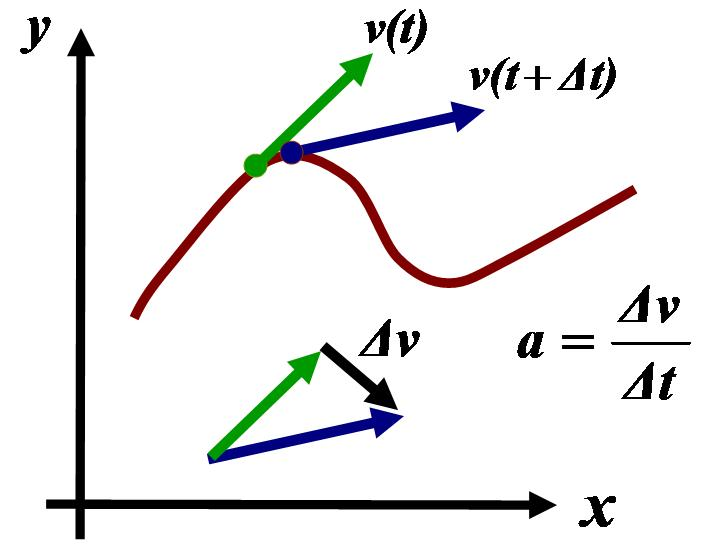# Graphs of acceleration, velocity, position

oneplusone
im confused with finding the max velocity , acceleration, and position.
Usually in these types of problems I'm given a function, and an interval and have to find the max acceleration or velocity.

Sometimes a negative number comes up which is greater in magnitude than the other numbers, and that is the answer. I don't get why. Can someone please explain?
Sorry if I'm being vague, i can't really explain it too well.

Mentor
I don't see what would be special about a negative number. Negative and positive are arbitrary anyway, you could measure in the other direction and all signs would get swapped.

Gold Member
Like mfb said, it's about directions.

Consider a horizontal line (which is 1-dimensional) and define a particular position, (i.e. point) as (0): all the positions (points) to the left of (0) will be negative, and all the positions (points) to the right of (0) will be positive.

• A negative position will mean a position to the left of (0), a positive position will mean a position to the right of (0).
• A negative velocity will mean motion to the left along the line, a positive velocity will mean motion to the right.
• A negative acceleration will mean acceleration to the left along the line, a positive acceleration will mean acceleration to the right.
The same applies for 2-dimensional and 3-dimensional space too. Velocity and acceleration (and forces etc.) are vector quantities. Vectors have both magnitude and direction. See Euclidean vector.